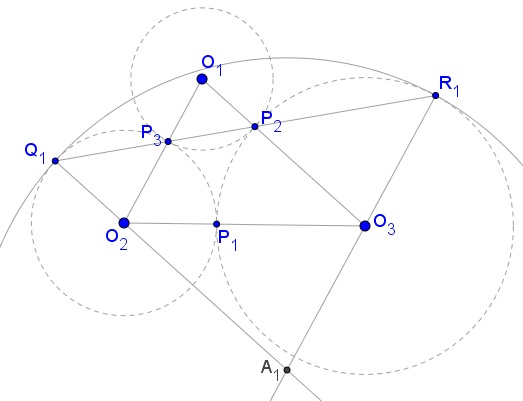Three Tangent Circles

Created with GeoGebra

Three circles (Oi), with centers at Oi, i = 1, 2, 3, touch pairwise externally. If i, j, k are three different indices obtained from 1, 2, 3 by a cyclic permutation, then Pk denotes the point of contact of (Oi) and (Oj). PiPj extended crosses again (Oi) in Qk and (Oj) in Rk. The circle (Ak) tangent to (Oi) in Qk and (Oj) in Rk have an interesting property.Three circles (Oi), with centers at Oi, i = 1, 2, 3, touch pairwise externally. If i, j, k are three different indices obtained from 1, 2, 3 by a cyclic permutation, then Pk denotes the point of contact of (Oi) and (Oj). PiPj extended crosses again (Oi) in Qk and (Oj) in Rk. Let ri denote the radius of circle (Oi).The center Ak of circle (Ak) lies at the intersection of lines CiQk and CjRk. One passes through Pj, the other through Pi. Note that, say Pj, is the center of similarity of (Oi) and (Ok). Therefore, triangles PjOkPi and PjOiQk are similar. Which makes lines OkPi and OiQk parallel. Thus, OkOj||OiAk. Similarly, OkOi||OjAk. The quadrilateral OiOkOjAk is a parallelogram. Which implies

AkOi = OjOk = rj + rk.

But the radius of circle (Ak) equals

AkQk = AkOi + OiQk = rj + rk + ri.

Which means that the radius of circle (Ak) is the sum of the radii of the given three circles. Since there is nothing special about the index k, all three circles A have the same radius and are, therefore, equal.

References

1. J. Hadamard, Leçons de géométrie élémentaire, tome I, 13e édition, reprint 1988, #322